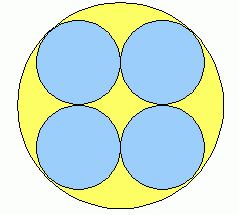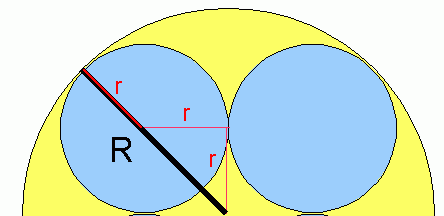SEARCH HOMEMath Central Quandaries & QueriesQuestion from Avaline, a student: Imagine that there are four small circles inscribed in a bigger circle. The 4 small circles are shaded. What is the ratio of the area of the shaded region to the area of the unshaded region?Hi Avaline.

Let's examine a diagram first:Now let's add a few dimensional lines to help analyze this figure:As you can see, the radius of the large circle is R and the radius of one shaded (blue) circle is r.

4πr2 : (πR2 - 4πr2)

This doesn't finish the problem, because we have two variables in there. Can you examine the lines I drew and come up with a relationship between r and R? Then you can substitute this in and the last variable will cancel out leaving you with a simple ratio.

Hope this helps,
Stephen La Rocque.Math Central is supported by the University of Regina and The Pacific Institute for the Mathematical Sciences.